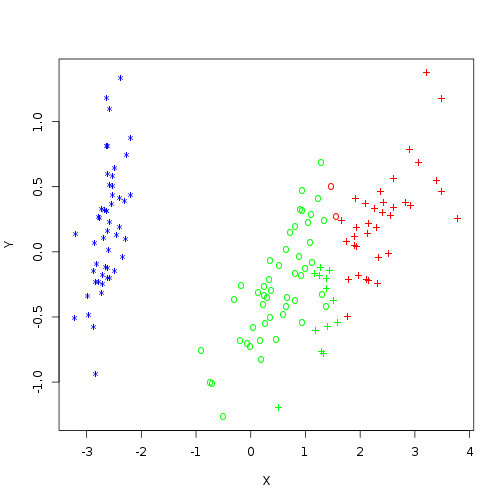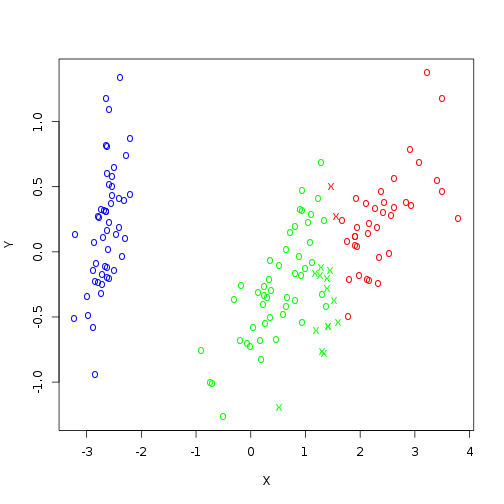# k-means clustering in R

We start by importing the famous Iris flower data-set. Because k-means is non-deterministic we also need to set our random seed to a constant so that we can reproduce the analysis.

``````data("iris") set.seed(321)
``````

Next we need perform kmeans using the data-set excluding the species column, which is the fifth column. We know that there are three clusters in this data-set (you usually don’t know the number of clusters), we are using at-most 1000 iterations, which is much higher than the conservative default of 10 iterations. However with modern hardware the difference will not be noticeable for a data-set this small.

``````iris.km <- kmeans(iris[, -5], 3, iter.max = 1000)
``````

We can then generate a table of the classifications like this.

``````tbl <- table(iris[, 5], iris.km\$cluster)
``````

| Actual | Cluster 1 | Cluster 2 | Cluster 3 |
| setosa | 0 | 0 | 50 |
| versicolor | 2 | 48 | 0 |
| virginica | 36 | 14 | 0 |

As you can see all the members of the setosa species end up in the third cluster, the majority of versicolor end up in the second cluster, leaving the majority of virginica in the first cluster.

Because our data is multivariate we need to translate it into a two dimensions using Multidimensional scaling.

``````iris.dist <- dist(iris[, -5]) iris.mds <- cmdscale(iris.dist)
``````

Once we have scaled points we can plot our points in 2 dimensional space. We now use colors and printing chars to indicate how they were classified.

``````c.chars <- c("*", "o", "+")[as.integer(iris\$Species)]
a.cols <- rainbow(3)[iris.km\$cluster]
plot(iris.mds, col = a.cols, pch = c.chars, xlab = "X", ylab = "Y")
``````We can also make a plot showing which classifications were correct.

``````corr <- iris.km\$cluster == 4 - as.integer(iris\$Species)
correct <- c("o", "x")[2 - corr]
plot(iris.mds, col = a.cols, pch = correct, xlab = "X", ylab = "Y")
``````William Rudenmalm is a european technologist passionate about the next big thing and the people building it. William is particularly interested in scaling engineering organizations and navigating trade offs of architecture and velocity. In the field of computer science, his expertise lies in distributed systems, scalability and machine learning. Among the technologies William is particularly excited about are Kubernetes, Rust, nats, Kafka and neo4j.